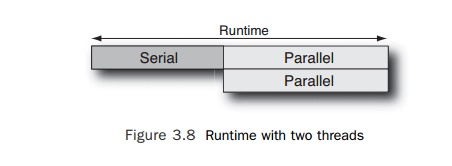# AmdahlŌĆÖs Law

AmdahlŌĆÖs law is the simplest form of a scaling law. The underlying assumption is that the performance of the parallel code scales with the number of threads. This is unrealistic, as we will discuss later, but does provide a basic starting point.

AmdahlŌĆÖs Law

AmdahlŌĆÖs law is the simplest form of a scaling law. The underlying assumption is that the performance of the parallel code scales with the number of threads. This is unrealistic, as we will discuss later, but does provide a basic starting point. If we assume that S repre-sents the time spent in serial code that cannot be parallelized and P represents the time spent in code that can be parallelized, then the runtime of the serial application is as follows:

Runtime = S + P

The runtime of a parallel version of the application that used N processors would take the following:It is probably easiest to see the scaling diagrammatically. In Figure 3.7, we represent the runtime of the serial portion of the code and the portion of the code that can be made to run in parallel as rectangles.If we use two threads for the parallel portion of the code, then the runtime of that part of the code will halve, and Figure 3.8 represents the resulting processor activity.If we were to use four threads to run this code, then the resulting processor activity would resemble Figure 3.9.There are a couple of things that follow from AmdahlŌĆÖs law. As the processor count increases, performance becomes dominated by the serial portion of the application. In the limit, the program can run no faster than the duration of the serial part, S. Another observation is that there are diminishing returns as the number of threads increases: At some point adding more threads does not make a discernible difference to the total runtime.

These two observations are probably best illustrated using the chart in Figure 3.10, which shows the parallel speedup over the serial case for applications that have various amounts of code that can be parallelized.If all the code can be made to run in parallel, the scaling is perfect; a code run with 18 threads will be 18x faster than the serial version of the code. However, it is surprising to see how fast scaling declines as the proportion of code that can be made to run in parallel drops. If 99% of the application can be converted to parallel code, the application would scale to about 15x the serial performance with 18 threads. At 95% serial, this would drop to about 10x the serial performance. If only half the application can be run in parallel, then the best that can be expected is for performance to double, and the code would pretty much attain that at a thread count of about 8.

There is another way of using AmdahlŌĆÖs law, and that is to look at how many threads an application can scale to given the amount of time it spends in code that can be parallelized.

Study Material, Lecturing Notes, Assignment, Reference, Wiki description explanation, brief detail
Multicore Application Programming For Windows, Linux, and Oracle Solaris : Identifying Opportunities for Parallelism : AmdahlŌĆÖs Law |